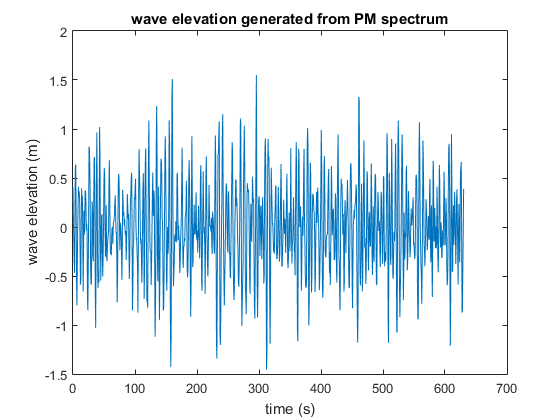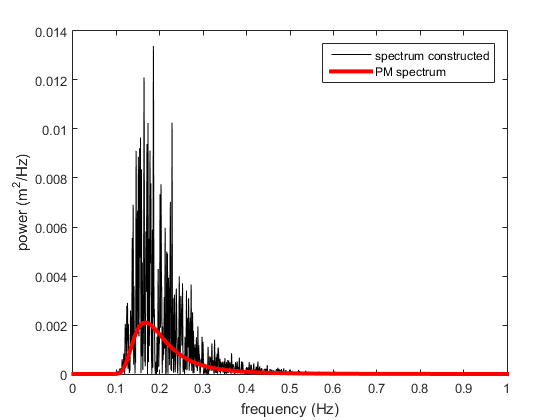# Pierson Moskowitz spectrum

Hello everyone,

I was trying to generate time series from a spectrum. In particular, i am generating wave elevation in time domain from PM spectrum (Pierson Moskowitz). In order to verify that i generate the correct time series, i should reconstruct the spectrum from the time series i have generated.

Please give your opinion regarding the reconstructed spectrum in comparison with the initial PM spectrum.

Here a figure for the time series generated from PM:Here a comparison between the reconstructed spectrum and Pm spectrum:I was thinking of a normalization problem.

Best Regards,

• Are you using the HydroDyn module of OpenFAST to generate the wave elevation time series from the PM spectra?
• Are you randomizing both the amplitude and phase of each wave component about the target PM spectra (`WaveNDAmp` = True in HydroDyn) or are you just randomizing the phase of each wave component, using the amplitude from the target PM spectra (`WaveNDAmp` = False) in HydroDyn.
• Are you resampling the data in any way or are the time step/length and frequency step/length consistent?

Best regards,

1 Like

Dear @Jason.Jonkman ,

• No i am not using HydroDyn module to generate time series. In fact, i am using MATLAB.

• i am randomizing the phase only. The phase is a uniformly distributed random variable varying between 0 and 2*pi

• In fact, i used the following parameters:
frequency step=1e-3 Hz;
time step=1e-2 seconds;
Number of samples = 63001
duration of the sample is 630 sec
sampling frequency is 100 Hz

• I did not understand what you mean by the time step/length and frequency step/length consistent.

Best Regards,